# Computing the local factors of an elliptic curve

The simplest nontrivial case of a zeta function is for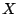an elliptic curve; in this case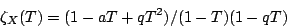for some integer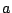, and the problem is simply to determine. Moreover, by the Weil conjectures,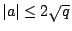.

Schoof [ MR 86e:11122] [ MR 97i:11070] introduced an algorithm for computing the zeta function of an elliptic curve over a finite field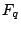which is polynomial time in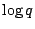. His strategy is to computemodulo enough small primes that the Chinese remainder theorem plus the Weil bound ontogether determineuniquely. Practical improvements to the algorithm were later introduced by Atkin and Elkies. The result is fairly efficient in practice, and many implementations are available (e.g., in the computer algebra package Magma).

In small characteristic, even more efficient methods are available. The algorithm of Satoh [ MR 2001j:11049] computes the zeta function of an ordinary elliptic curve over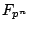, for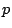prime, in time polynomial inand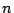, essentially by computingmodulo a suitably high power of. (The restriction to ordinary elliptic curves is not a big problem, as the supersingular case is quite easy to handle, even by hand.) Thus it is not useful for fields of large characteristic. However, it is more efficient than Schoof's algorithm for fields of small characteristic. Satoh's algorithm was originally limited to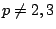; later authors have removed this restriction, e.g. Fouquet, Gaudry and Mestre [1 801 223] and Skjernaa [B. Skjernaa, Satoh point counting in characteristic 2, preprint].

Satoh's algorithm involves iteratively computing the Serre-Tate canonical lift of the given elliptic curve. It may be possible to streamline the calculation by retaining the iteration while omitting some irrelevant data about the canonical lift. An example of is the AGM method of Gaudry, Harley and Mestre (Eurocrypt 2001 rump session), which is limited to characteristic 2 but outperforms all known algorithms in that case.

Back to the main index for L-functions and Random Matrix Theory.